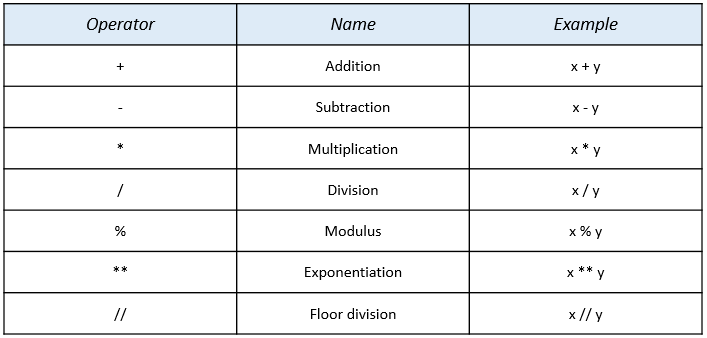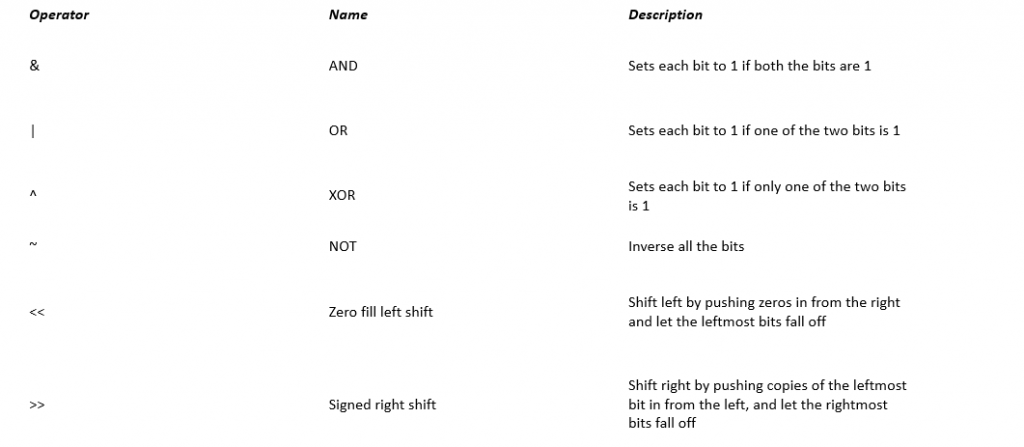# Lesson 2 - Operators in Python

• Python supports the following groups of operators. These are used to perform various operations on values and variables.

• Arithmetic operators
• Assignment operators
• Comparison operators
• Logical operators
• Identity operators
• Membership operators
• Bitwise operators

Arithmetic operators
Arithmetic operators are used with numeric values to perform common mathematical operations like addition, subtraction, etcExample:

x=10; y=15
print(x+y)  gives output as 25

Assignment operators

Assignment operators are used to assign values to variablesPython Comparison Operators

Comparison operators are used to compare two values or two variables:Python Logical Operators

Logical operators are used to combine conditional statements:Python Identity Operators

Identity operators:  is  and is not.  They are used to compare the objects to check if they are actually the same object, with the same memory location:Python Membership Operators

in and not in are the membership operators. These are used to test whether a value or variable is in a sequence.Python Bitwise Operators

Bitwise operators are used to compare (binary) numbers. They act on bits and perform the bit-by-bit operations.